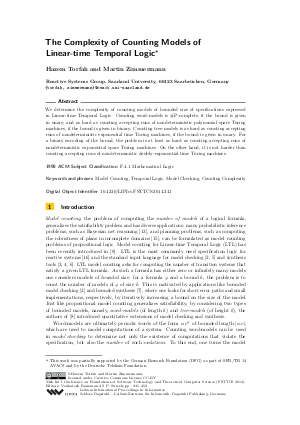Document# The Complexity of Counting Models of Linear-time Temporal Logic

### Authors Hazem Torfah, Martin Zimmermann## File

LIPIcs.FSTTCS.2014.241.pdf
• Filesize: 0.49 MB
• 12 pages

## Cite As

Hazem Torfah and Martin Zimmermann. The Complexity of Counting Models of Linear-time Temporal Logic. In 34th International Conference on Foundation of Software Technology and Theoretical Computer Science (FSTTCS 2014). Leibniz International Proceedings in Informatics (LIPIcs), Volume 29, pp. 241-252, Schloss Dagstuhl - Leibniz-Zentrum für Informatik (2014)
https://doi.org/10.4230/LIPIcs.FSTTCS.2014.241

## Abstract

We determine the complexity of counting models of bounded size of specifications expressed in Linear-time Temporal Logic. Counting word-models is #P-complete, if the bound is given in unary, and as hard as counting accepting runs of nondeterministic polynomial space Turing machines, if the bound is given in binary. Counting tree-models is as hard as counting accepting runs of nondeterministic exponential time Turing machines, if the bound is given in unary. For a binary encoding of the bound, the problem is at least as hard as counting accepting runs of nondeterministic exponential space Turing machines. On the other hand, it is not harder than counting accepting runs of nondeterministic doubly-exponential time Turing machines.
##### Keywords
• Model Counting
• Temporal Logic
• Model Checking
• Counting Complexity

## Metrics

• Access Statistics
• Total Accesses (updated on a weekly basis)
0